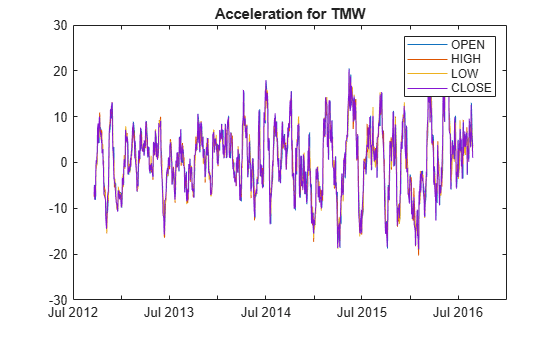# tsmom

Momentum between times

## Syntax

``momentum = tsmom(Data)``
``momentum = tsmom(___,Name,Value)``

## Description

example

````momentum = tsmom(Data)` calculates the momentum of a data series with time distance of n periods.```

example

````momentum = tsmom(___,Name,Value)` adds optional name-value pair arguments. ```

## Examples

collapse all

Load the file `SimulatedStock.mat`, which provides a timetable (`TMW`) for financial data for TMW stock.

```load SimulatedStock.mat TMW.Volume = []; % remove VOLUME field momentum = tsmom(TMW); plot(momentum.Time,momentum.Variables) legend('OPEN','HIGH','LOW','CLOSE') title('Acceleration for TMW')```## Input Arguments

collapse all

Data with high, low, open, close information, specified as a vector, matrix, table, or timetable. For vector input, `Data` is a column vector. For matrix input, `Data` is an `M`-by-`N` column oriented matrix. Timetables and tables with `M` rows can contain variables named `'High'`, `'Low'`, `'Open'`, and `'Close'` (case insensitive).

Data Types: `double` | `table` | `timetable`

### Name-Value Arguments

Specify optional pairs of arguments as `Name1=Value1,...,NameN=ValueN`, where `Name` is the argument name and `Value` is the corresponding value. Name-value arguments must appear after other arguments, but the order of the pairs does not matter.

Before R2021a, use commas to separate each name and value, and enclose `Name` in quotes.

Example: `momentum = tsmom(TMW,'NumPeriods',15)`

Period difference for momentum, specified as the comma-separated pair consisting of `'NumPeriods'` and a scalar positive integer.

Data Types: `double`

## Output Arguments

collapse all

Momentum series, returned with the same number of rows (`M`) and the same type (matrix, table, or timetable) as the input `Data`.